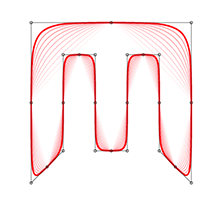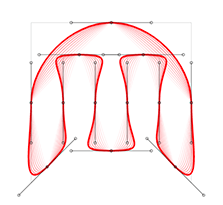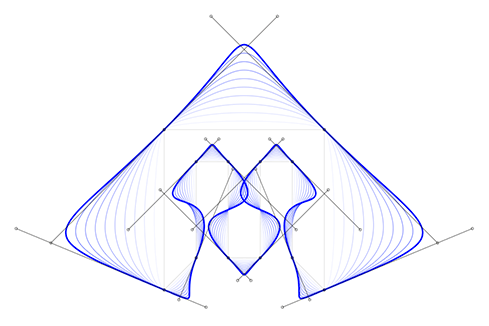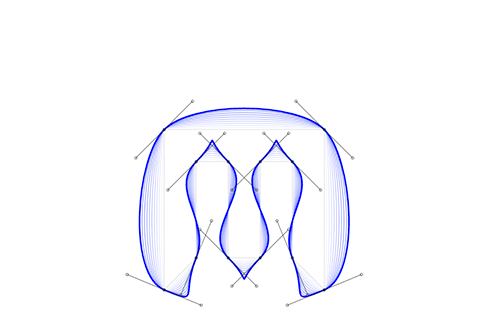Options
All
• Public
• Public/Protected
• All

# @thi.ng/geom-splinesThis project is part of the @thi.ng/umbrella monorepo.

nD cubic & quadratic curve analysis, conversion, interpolation, splitting, resampling.

## Installation

``yarn add @thi.ng/geom-splines``

## Usage examples

### Polygon to cubic curve conversion

Currently, there're two ways to convert a polygon into a sequence of cubic curve segments. Both modes support customizable curve tightness.

Furthermore, both conversion modes can be instructed to use uniformly scaled control point tangents: In uniform mode, the tangents have a uniform, user defined length, resulting in equidistant control points for each poly vertex. In non-uniform mode, each tangent is scaled by the length of its parent poly edge.

#### Poly vertices as control points

In this mode the curve always goes through the midpoints each polygon edge, with the original polygon vertices being used to compute control points.

Proportional tangent scale Uniform tangent scale#### Poly vertices as break points

In this mode the curve always goes through the original polygon vertices and additional control points are created via symmetric tangents at each poly vertex. The tangents themselves are computed via the bisector of each vertex corner, taking into the convexity of each poly vertex.

Proportional tangent scale Uniform tangent scale``import * as gs from "@thi.ng/geom-splines";``

## Authors

• Karsten Schmidt